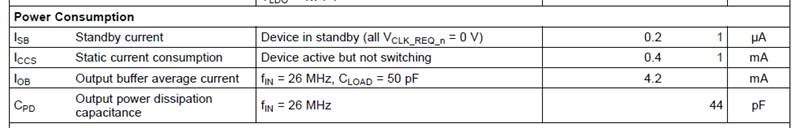If you have a related question, please click the "Ask a related question" button in the top right corner. The newly created question will be automatically linked to this question.

# CDC3RL02: power consumption calc

Part Number: CDC3RL02

Hi Team,

Does the following table show the total power consumption of the device? How to calculate the total power consumption of the device accurately?• Hi Gene,

The power dissipation outside of standby is Vcc * (Iccs + Iob * n) where n is the number of clock outputs requested.

Iob = Fout * Vpp_out * (Cpd + Cload). As an example, the output buffer average current of 4.2mA = 26MHz * 1.8V * (44pF + 50pF) = about 4.4mA. Since the output power dissipation capacitance is only given as a maximum value, chances are this value is slightly smaller in practice, but this can give you an upper bound on current consumption for active buffers.

Iccs is not characterized in any plots over temperature, so my recommendation is to use the typical value at typical temperature and the max value at max temperature.

Note also there are several figures (2-5) in the datasheet which help to predict power consumption.

Regards,